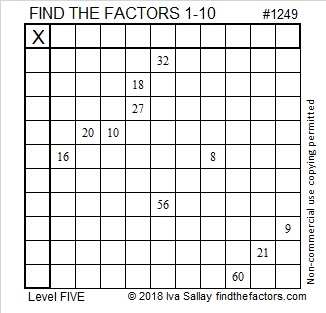# 1249 and Level 5

Level 5 puzzles can be a little tricky, but if you think about all the factors for the given clues and use logic, you should be able to solve this one. Even if the puzzle tricks you, don’t give up!Print the puzzles or type the solution in this excel file: 10-factors-1242-1250

Now I’ll share some facts about the number 1249:

• 1249 is a prime number.
• Prime factorization: 1249 is prime.
• The exponent of prime number 1249 is 1. Adding 1 to that exponent we get (1 + 1) = 2. Therefore 1249 has exactly 2 factors.
• Factors of 1249: 1, 1249
• Factor pairs: 1249 = 1 × 1249
• 1249 has no square factors that allow its square root to be simplified. √1249 ≈ 35.34119

How do we know that 1249 is a prime number? If 1249 were not a prime number, then it would be divisible by at least one prime number less than or equal to √1249 ≈ 35.3. Since 1249 cannot be divided evenly by 2, 3, 5, 7, 11, 13, 17, 19, 23, 29 or 31, we know that 1249 is a prime number.1249 is the sum of three consecutive prime numbers:
409 + 419 + 421 = 1249

1249 is the sum of two squares:
32² + 15² = 1249

1249 is the hypotenuse of a Pythagorean triple:
799-960-1249 calculated from 32² – 15², 2(32)(15), 32² + 15²

Here’s another way we know that 1249 is a prime number: Since its last two digits divided by 4 leave a remainder of 1, and 32² + 15² = 1249 with 32 and 15 having no common prime factors, 1249 will be prime unless it is divisible by a prime number Pythagorean triple hypotenuse less than or equal to √1249 ≈ 35.3. Since 1249 is not divisible by 5, 13, 17, or 29, we know that 1249 is a prime number.